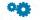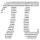# Circle - high school - examples

1. The diagram 2The diagram shows a cone with slant height 10.5cm. If the curved surface area of the cone is 115.5 cm2. Calculate correct to 3 significant figures: *Base Radius *Height *Volume of the cone
2. Find parametersFind parameters of the circle in the plane - coordinates of center and radius: ?
3. Spherical cap 4What is the surface area of a spherical cap, the base diameter 20 m, height 2.5 m? Calculate using formula.
4. Circle chordWhat is the length d of the chord circle of diameter 36 m, if distance from the center circle is 16 m?
5. RectangleThe rectangle is 11 cm long and 45 cm wide. Determine the radius of the circle circumscribing rectangle.
6. ClockHow many times a day hands on a clock overlap?
7. Cone A2VSurface of cone in the plane is a circular arc with central angle of 126° and area 415 cm2. Calculate the volume of a cone.
8. RectangleIn rectangle with sides 10 and 8 mark the diagonal. What is the probability that a randomly selected point within the rectangle is closer to the diagonal than to any side of the rectangle?
9. Square and circlesSquare with sides 61 mm is circumscribed and inscribed with circles. Determine the radiuses of both circles.
10. CirclesThe areas of the two circles are in the ratio 2:20. The larger circle has diameter 20. Calculate the radius of the smaller circle.
11. CylindersArea of the side of two cylinders is same rectangle of 50 cm × 11 cm. Which cylinder has a larger volume and by how much?
12. Circle arcCircle segment has a circumference of 135.26 dm and 2096.58 dm2 area. Calculate the radius of the circle and size of central angle.
13. RhombusIt is given a rhombus of side length a = 29 cm. Touch points of inscribed circle divided his sides into sections a1 = 14 cm and a2 = 15 cm. Calculate the radius r of the circle and the length of the diagonals of the rhombus.
14. Rhombus and inscribed circleIt is given a rhombus with side a = 6 cm and the radius of the inscribed circle r = 2 cm. Calculate the length of its two diagonals.
15. GearTwo gears, fit into each other, has transfer 2:3. Centres of gears are spaced 82 cm. What are the radii of the gears?
16. TangentsTo circle with a radius of 41 cm from the point R guided two tangents. The distance of both points of contact is 16 cm. Calculate the distance from point R and circle centre.
17. RadiansConvert 270° to radians. Write result as multiple of number π.
18. Circular poolThe base of pool is circle with a radius r = 10 m excluding circular segment that determines chord length 10 meters. Pool depth is h = 2m. How many hectoliters of water can fit into the pool?
19. DescribedCalculate perimeter of the circle described by a triangle with sides 478, 255, 352.
20. Arc and segmentCalculate the length of circular arc l, area of the circular arc S1 and area of circular segment S2. Radius of the circle is 33 and corresponding angle is ?.

Do you have an interesting mathematical example that you can't solve it? Enter it, and we can try to solve it.

To this e-mail address, we will reply solution; solved examples are also published here. Please enter e-mail correctly and check whether you don't have a full mailbox.